# St. Vincent de Paul Home Learning W1 5.1.21

4 Jan 2021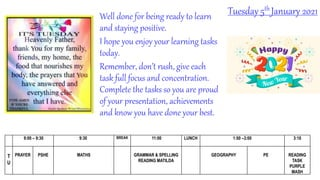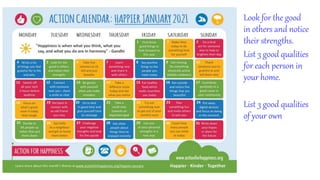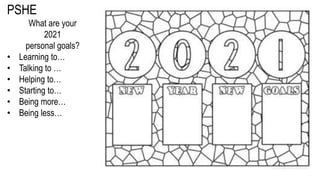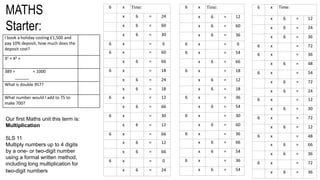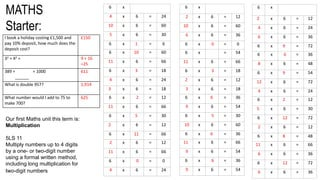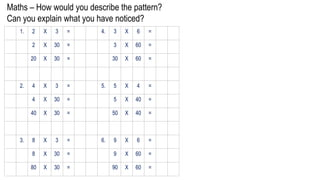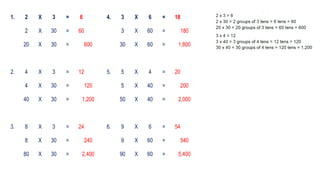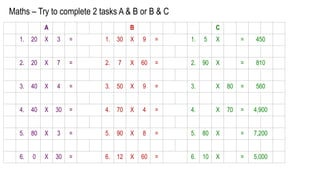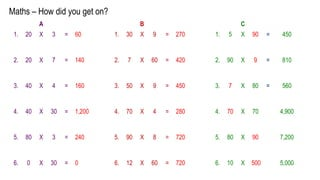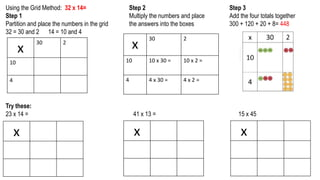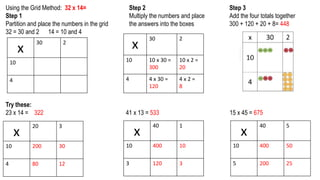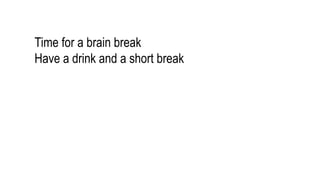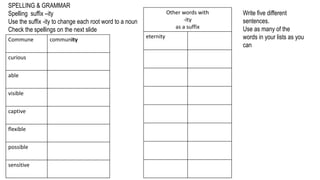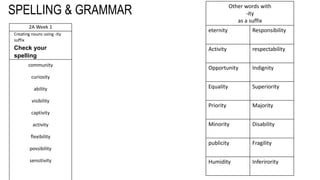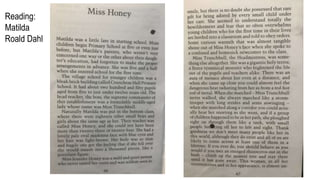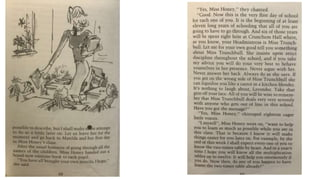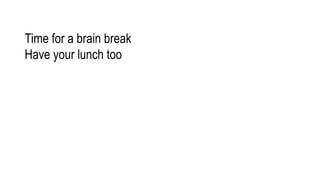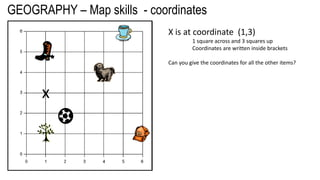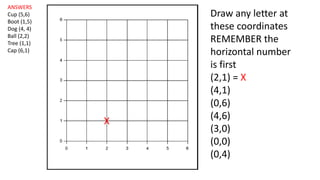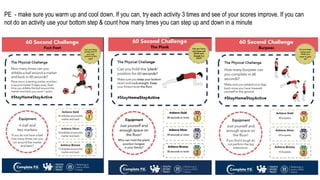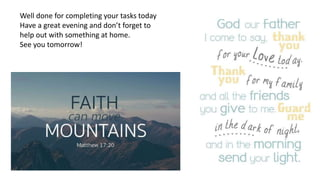1 sur 22

### St. Vincent de Paul Home Learning W1 5.1.21

• 1. Tuesday 5th January 2021 9:00 – 9:30 9:30 BREAK 11:00 LUNCH 1:00 –3:00 3:10 T U PRAYER PSHE MATHS GRAMMAR & SPELLING READING MATILDA GEOGRAPHY PE READING TASK PURPLE MASH Well done for being ready to learn and staying positive. I hope you enjoy your learning tasks today. Remember, don’t rush, give each task full focus and concentration. Complete the tasks so you are proud of your presentation, achievements and know you have done your best.
• 2. Look for the good in others and notice their strengths. List 3 good qualities for each person in your home. List 3 good qualities of your own
• 3. PSHE What are your 2021 personal goals? • Learning to… • Talking to … • Helping to… • Starting to… • Being more… • Being less…
• 4. MATHS Starter: I book a holiday costing £1,500 and pay 10% deposit, how much does the deposit cost? 32 + 42 = 389 + = 1000 ______ What is double 957? What number would I add to 75 to make 700? Our first Maths unit this term is: Multiplication 5LS 11 Multiply numbers up to 4 digits by a one- or two-digit number using a formal written method, including long multiplication for two-digit numbers 6 x Time: x 6 = 24 x 6 = 60 x 6 = 30 6 x = 6 6 x = 60 x 6 = 66 6 x = 18 x 6 = 24 x 6 = 18 6 x = 12 x 6 = 66 6 x = 30 x 6 = 12 6 x = 66 x 6 = 12 x 6 = 66 6 x = 0 x 6 = 24 6 x Time: x 6 = 12 x 6 = 60 x 6 = 36 6 x = 0 6 x = 54 x 6 = 66 6 x = 18 x 6 = 12 x 6 = 18 6 x = 36 x 6 = 54 6 x = 30 x 6 = 60 6 x = 36 x 6 = 66 x 6 = 54 6 x = 36 x 6 = 54 6 x Time: x 6 = 12 x 6 = 24 x 6 = 36 6 x = 72 6 x = 36 x 6 = 48 6 x = 54 x 6 = 72 x 6 = 24 6 x = 12 x 6 = 30 6 x = 72 x 6 = 12 6 x = 48 x 6 = 66 x 6 = 36 6 x = 72 x 6 = 36
• 5. MATHS Starter: I book a holiday costing £1,500 and pay 10% deposit, how much does the deposit cost? £150 32 + 42 = 9 + 16 =25 389 + = 1000 ______ 611 What is double 957? 1,914 What number would I add to 75 to make 700? 625 Our first Maths unit this term is: Multiplication 5LS 11 Multiply numbers up to 4 digits by a one- or two-digit number using a formal written method, including long multiplication for two-digit numbers 6 x 4 x 6 = 24 10 x 6 = 60 5 x 6 = 30 6 x 1 = 6 6 x 10 = 60 11 x 6 = 66 6 x 3 = 18 4 x 6 = 24 3 x 6 = 18 6 x 2 = 12 11 x 6 = 66 6 x 5 = 30 2 x 6 = 12 6 x 11 = 66 2 x 6 = 12 11 x 6 = 66 6 x 0 = 0 4 x 6 = 24 6 x 2 x 6 = 12 10 x 6 = 60 6 x 6 = 36 6 x 0 = 0 6 x = 54 11 x 6 = 66 6 x 3 = 18 2 x 6 = 12 3 x 6 = 18 6 x 6 = 36 9 x 6 = 54 6 x 5 = 30 10 x 6 = 60 6 x 6 = 36 11 x 6 = 66 9 x 6 = 54 6 x 6 = 36 9 x 6 = 54 6 x 2 x 6 = 12 4 x 6 = 24 6 x 6 = 36 6 x 9 = 72 6 x 6 = 36 8 x 6 = 48 6 x 9 = 54 12 x 6 = 72 4 x 6 = 24 6 x 2 = 12 5 x 6 = 30 6 x 12 = 72 2 x 6 = 12 6 x 8 = 48 11 x 6 = 66 6 x 6 = 36 6 x 12 = 72 6 x 6 = 36
• 6. 1. 2 X 3 = 4. 3 X 6 = 2 X 30 = 3 X 60 = 20 X 30 = 30 X 60 = 2. 4 X 3 = 5. 5 X 4 = 4 X 30 = 5 X 40 = 40 X 30 = 50 X 40 = 3. 8 X 3 = 6. 9 X 6 = 8 X 30 = 9 X 60 = 80 X 30 = 90 X 60 = Maths – How would you describe the pattern? Can you explain what you have noticed?
• 7. 1. 2 X 3 = 6 4. 3 X 6 = 18 2 X 30 = 60 3 X 60 = 180 20 X 30 = 600 30 X 60 = 1,800 2. 4 X 3 = 12 5. 5 X 4 = 20 4 X 30 = 120 5 X 40 = 200 40 X 30 = 1,200 50 X 40 = 2,000 3. 8 X 3 = 24 6. 9 X 6 = 54 8 X 30 = 240 9 X 60 = 540 80 X 30 = 2,400 90 X 60 = 5,400
• 8. A B C 1. 20 X 3 = 1. 30 X 9 = 1. 5 X = 450 2. 20 X 7 = 2. 7 X 60 = 2. 90 X = 810 3. 40 X 4 = 3. 50 X 9 = 3. X 80 = 560 4. 40 X 30 = 4. 70 X 4 = 4. X 70 = 4,900 5. 80 X 3 = 5. 90 X 8 = 5. 80 X = 7,200 6. 0 X 30 = 6. 12 X 60 = 6. 10 X = 5,000 Maths – Try to complete 2 tasks A & B or B & C
• 9. Maths – How did you get on? A B C 1. 20 X 3 = 60 1. 30 X 9 = 270 1. 5 X 90 = 450 2. 20 X 7 = 140 2. 7 X 60 = 420 2. 90 X 9 = 810 3. 40 X 4 = 160 3. 50 X 9 = 450 3. 7 X 80 = 560 4. 40 X 30 = 1,200 4. 70 X 4 = 280 4. 70 X 70 4,900 5. 80 X 3 = 240 5. 90 X 8 = 720 5. 80 X 90 7,200 6. 0 X 30 = 0 6. 12 X 60 = 720 6. 10 X 500 5,000
• 10. Using the Grid Method: 32 x 14= Step 1 Partition and place the numbers in the grid 32 = 30 and 2 14 = 10 and 4 x 30 2 10 4 Step 2 Multiply the numbers and place the answers into the boxes x 30 2 10 10 x 30 = 10 x 2 = 4 4 x 30 = 4 x 2 = Step 3 Add the four totals together 300 + 120 + 20 + 8= 448 x x x Try these: 23 x 14 = 41 x 13 = 15 x 45
• 11. Using the Grid Method: 32 x 14= Step 1 Partition and place the numbers in the grid 32 = 30 and 2 14 = 10 and 4 x 30 2 10 4 Step 2 Multiply the numbers and place the answers into the boxes x 30 2 10 10 x 30 = 300 10 x 2 = 20 4 4 x 30 = 120 4 x 2 = 8 Step 3 Add the four totals together 300 + 120 + 20 + 8= 448 x 20 3 10 200 30 4 80 12 x 40 1 10 400 10 3 120 3 x 40 5 10 400 50 5 200 25 Try these: 23 x 14 = 322 41 x 13 = 533 15 x 45 = 675
• 12. Time for a brain break Have a drink and a short break
• 13. SPELLING & GRAMMAR Spelling suffix –ity Use the suffix -ity to change each root word to a noun Check the spellings on the next slide Commune community curious able visible captive flexible possible sensitive Other words with -ity as a suffix eternity Write five different sentences. Use as many of the words in your lists as you can
• 14. SPELLING & GRAMMAR 2A Week 1 Creating nouns using -ity suffix Check your spelling community curiosity ability visibility captivity activity flexibility possibility sensitivity Other words with -ity as a suffix eternity Responsibility Activity respectability Opportunity Indignity Equality Superiority Priority Majority Minority Disability publicity Fragility Humidity Inferirority
• 15. Reading: Matilda Roald Dahl
• 17. Time for a brain break Have your lunch too
• 18. GEOGRAPHY – Map skills - coordinates x X is at coordinate (1,3) 1 square across and 3 squares up Coordinates are written inside brackets Can you give the coordinates for all the other items?
• 19. ANSWERS Cup (5,6) Boot (1,5) Dog (4, 4) Ball (2,2) Tree (1,1) Cap (6,1) Draw any letter at these coordinates REMEMBER the horizontal number is first (2,1) = X (4,1) (0,6) (4,6) (3,0) (0,0) (0,4) X
• 20. PE - make sure you warm up and cool down. If you can, try each activity 3 times and see of your scores improve. If you can not do an activity use your bottom step & count how many times you can step up and down in a minute
• 21. READING There is a reading activity set on Purple Mash (2Do) A chapter book called A Bridge on Fire Each day there will be a chapter to read or a task to do related to the book
• 22. Well done for completing your tasks today Have a great evening and don’t forget to help out with something at home. See you tomorrow!